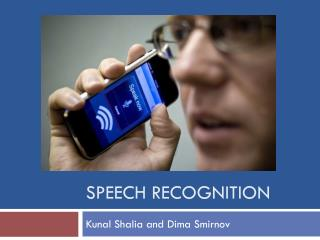DownloadDownload PresentationSpeech recognition

# Speech recognition

Download Presentation## Speech recognition

- - - - - - - - - - - - - - - - - - - - - - - - - - - E N D - - - - - - - - - - - - - - - - - - - - - - - - - - -
##### Presentation Transcript

1. Speech recognition KunalShaliaand Dima Smirnov

2. What is Speech Recognition? • Speech Recognition is the ability of a machine or program to identify words and phrases in spoken language and convert them to a machine readable format. • Speech Recognition vs. Voice Recognition

3. Speech Recognition Demonstration

4. Early Automatic SR Systems • Based on the theory of acoustic phonetics • Describes how phonetic elements are realized in speech • Compared input speech to reference patterns • Trajectories along the first and second formant frequencies for the numbers 1 through 9 and “oh”: • Used in the first speech recognizer built by Bell Laboratories in 1952

5. The Development of SR • 1950s • RCA Laboratories – recognizing 10 syllables spoken by a single speaker • MIT Lincoln Lab – speaker-independent 10-vowel recognition • 1960s • Kyoto University – speech segmenter • University College – first to use a statistical model of allowable phoneme sequences in the English language • RCA Laboratories – non-uniform time scale instead of speech segmentation • 1970s • Carnegie Mellon – graph search based on a beam algorithm

6. The Two Schools of SR • Two schools of applicability of ASR for commercial applications were developed in the 1970s • IBM • Speaker-dependent • Converted sentences into letters and words • Transcription - focus on the probability associated with the structure of the language model • N-gram model • AT&T • Speaker-independent • Emphasis on an acoustic model over language model

7. Markov Models • A stochastic model where each state depends only on the previous state in time. • The simplest Markov Model is the Markov chain which undergoes transitions from one state to the other through a random process. • Markov Property

8. Hidden Markov Models • A Hidden Markov Model (HMM) is a Markov Model using the Markov Property with unobserved (hidden) states. • In a Markov Model the states are directly visible to the observer, while in an HMM the state is not directly visible but the output ,which is dependent on the state, is visible.

9. Elements of a HMM • There are a finite number of N states, and each state possesses some measurable, distinctive properties. • At each clock time T, a new state is entered based upon a transition probability distribution which depends on the previous state(Markovian property) • After each transition, an observation output symbol is produced according to the probability distribution of the state.

10. Urn and Ball Example • We assume that there are N glass urns in room. • In each urn there is a large quantity of colored balls and M distinct colors. • A gene is in the room and randomly chooses the initial urn. • Then a ball is chosen at random, its color recorded, and then the ball is replaced in the same urn. • A new urn is selected according to a random procedure associated with the current urn.

11. Urn and Ball Example • Each state corresponds to a specific urn • Color probability is defined for each state (hidden)

12. Coin Toss Example • You are in a room with a barrier and you cannot see what is happening on the other side. • On the other side another person is performing a coin(or multiple coin) tossing experiment. • You wont know what is happening, but will receive the results of each coin flip. Thus a sequence of HIDDEN coin tosses are performed and you can only observe the results.

13. One coin toss

14. Two coins being tossed

15. Three coins being tossed

16. HMM Notation

17. The Three Problems for HMM • 1. Given the observation sequence O = (o1 . . . oT ), and a model λ = (A, B, π), how do we eﬃciently compute P (O|λ), the probability of the observation sequence given the model? • 2. Given the observation sequence O = (o1 . . . oT ), and a model λ = (A, B, π), how do we choose a corresponding sequence q = (q1 . . . qT ) that is optimal in some sense (i.e., best “explains” the observations)? • 3. How do we adjust the model parameters λ = (A, B, π) to maximize P (O|λ)?

18. 3 types of HMM • Ergodic Model • Left to Right Model • Parallel Left to Right Model

19. Ergodic Model • In an ergodic model it is possible to reach any state from any other state.

20. Left to Right (Bakis) Model • As time increases, the state index increases or stays the same

21. Parallel Right to Left Model • A left to right model where there are several paths through the states.

22. HMM in SR • 1980s – shift to rigorous statistical framework • HMM can model the variability in speech • Use Markov chains to represent linguistic structure and the set of probability distributions • Baum-Welch Algorithm to find unknown parameters • Hidden Markov Model merged with finite-state network

23. Speech Recognition Today • Developments in algorithms and data storage models have allowed more efficient methods of storing larger vocabulary bases • Modern Applications • Military • Health care • Telephony • Computing